# Precalculus : Fundamental Trigonometric Identities

## Example Questions

1 2 3 4 5 7 Next →

### Example Question #61 : Fundamental Trigonometric Identities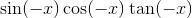Possible Answers: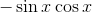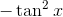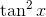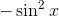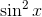Correct answer:Explanation:

Using these basic identities: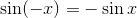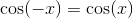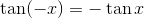we find the original expression to be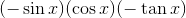which simplifies to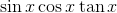.

Further simplifying: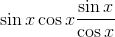The cosines cancel, giving us### Example Question #62 : Fundamental Trigonometric Identities

Which of the following is the best answer for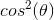?

Possible Answers: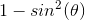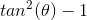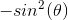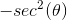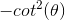Correct answer:Explanation:

Write the Pythagorean identity.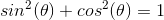Substract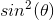from both sides.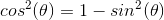The other answers are incorrect.

### Example Question #63 : Fundamental Trigonometric Identities

Express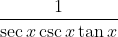in terms of only sines and cosines.

Possible Answers: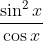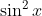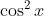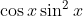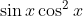Correct answer:Explanation:

The correct answer is. Begin by substituting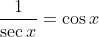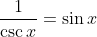, and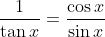. This gives us: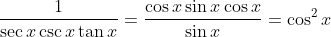.

### Example Question #171 : Trigonometric Functions

Express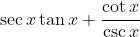in terms of only sines and cosines.

Possible Answers: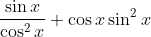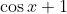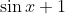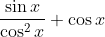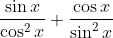Correct answer:Explanation:

To solve this problem, use the identities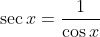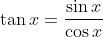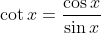, and. Then we get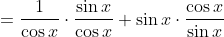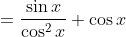1 2 3 4 5 7 Next →

### All Precalculus Resources